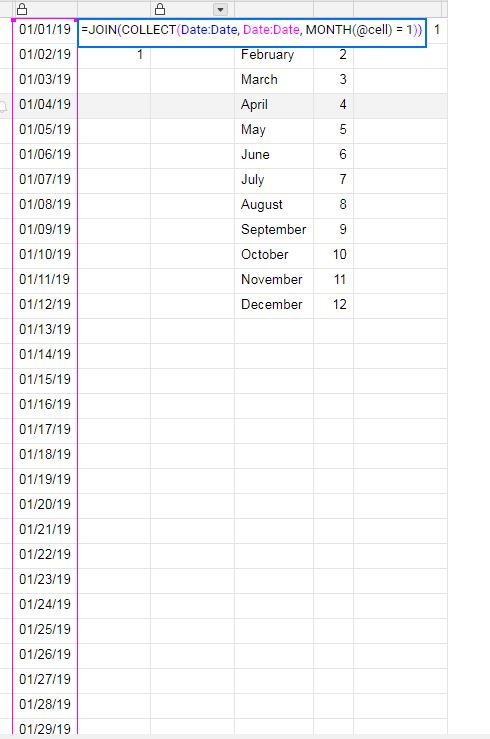or Explore Discussions

# Month Formula

07/31/19 Edited 12/09/19

Having some issues with the month formula popping up errors when being used as a reference for a range in the collect and countif/countifs functions

The below formula produces an error

=JOIN(COLLECT(Date:Date, Date:Date, MONTH(@cell) = 1))

The below formula returns a 1 even though every day in january is included in the Date column.

=Count(COLLECT(Date:Date, Date:Date, MONTH(@cell) = 1))

The simplest version of what I am trying to do is below, but it also produces an error

=countif(date:date,month(@cell)=1)

I've attached a picture below. Is anyone else having this issue? Anyone come up with a workaround?Popular Tags:

• If you have any blanks or cells that do not have a date in them, you will get an error. To account for these cells, wrap the MONTH function in an IFERROR to generate a 0 (zero) for a cell that would otherwise throw an error. Because it is replacing the error with 0 and there is no month 0, these cells will no longer throw the error and will not be counted in the formula.

=JOIN(COLLECT(Date:Date, Date:Date, IFERROR(MONTH(@cell), 0) = 1))

=COUNTIFS(Date:Date, IFERROR(MONTH(@cell), 0) = 1)

• kind of stupid that is needed... if the cell is blank the month isn't equal to 1. I would have never thought of that. Thanks

• The error is coming from the MONTH function itself. The MONTH function is looking for a date. Referencing a blank cell is the same as saying

=MONTH()

or a cell with "abc"

=MONTH("abc")

Which obviously will not work.

With the MONTH function throwing an error, the formula as a whole will break and in turn throw an error.

.

My explanation using "Month 0" isn't entirely accurate.

The MONTH function produces a numerical value which is what we are comparing to the number 1. The IFERROR is telling the formula that if the MONTH function throws an error (as explained above), just produce the number 0.

Since 0 is not equal to 1 but is still a numerical value that can be compared to other numerical values, it allows the rest of the formula to continue working while excluding it from the count.

The reason I use the number 0 is because you will never look for month (or year for that matter) 0.